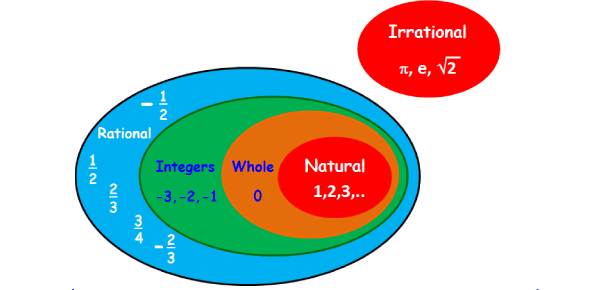# Real Number System Test Quiz!

19 Questions | Total Attempts: 177Settings.

• 1.
What is the best classification for -4?
• A.

integer, rational number, real number

• B.

irrational number, real number

• C.

Whole number, integer, real number

• D.

Rational number, real number

• 2.
Which of these sets of numbers contains all rational numbers?
• A.

π ,1,2, -13

• B.

-3.0541... , 99, 0.14363

• C.

-6, − 225, 4,7,8

• D.

21, 0.75, 0

• 3.
Given the following set of numbers, circle each irrational number (there may be more than one).
• A.

23

• B.

3

• C.

3.14

• D.

0

• E.

-6.5555

• F.

4/9

• G.

-2

• H.

π

• 4.
−5 is a rational number.
• A.

True

• B.

False

• 5.
0 is an integer.
• A.

True

• B.

False

• 6.
Square Root of 16 is a natural number.
• A.

True

• B.

False

• 7.
−3. 25 is an integer.
• A.

True

• B.

False

• 8.
Square Root of 8 is rational.
• A.

True

• B.

False

• 9.
Square Root of 7 is a Real number.
• A.

True

• B.

False

• 10.
18 is a whole number.
• A.

True

• B.

False

• 11.
− 3/2 is an integer.
• A.

True

• B.

False

• 12.
2.434434443… is a rational number.
• A.

True

• B.

False

• 13.
6.57 is an integer.
• A.

True

• B.

False

• 14.
5. 7777 is rational.
• A.

True

• B.

False

• 15.
All fractions are rational numbers.
• A.

True

• B.

False

• 16.
All integers are whole numbers.
• A.

True

• B.

False

• 17.
All irrational numbers are Real numbers.
• A.

True

• B.

False

• 18.
All negative numbers are integers.
• A.

True

• B.

FalseBack to top